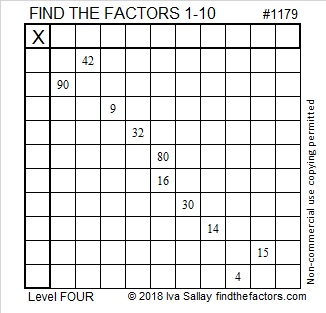# 1179 and Level 4

80 and 16 have just one common factor that will put only numbers from 1 to 10 in the first column and in the top row. Put those factors where they belong and use logic to figure out where to put the rest.Print the puzzles or type the solution in this excel file: 10-factors-1174-1186

Now I tell you what I’ve learned about the number 1179:

• 1179 is a composite number.
• Prime factorization: 1179 = 3 × 3 × 131, which can be written 1179 = 3² × 131
• The exponents in the prime factorization are 2 and 1. Adding one to each and multiplying we get (2 + 1)(1 + 1) = 3 × 2  = 6. Therefore 1179 has exactly 6 factors.
• Factors of 1179: 1, 3, 9, 131, 393, 1179
• Factor pairs: 1179 = 1 × 1179, 3 × 393, or 9 × 131
• Taking the factor pair with the largest square number factor, we get √1179 = (√9)(√131) = 3√131 ≈ 34.33657I like the way 1179 looks in a couple other bases:
It’s 2233 in BASE 8 because 2(8³ + 8²) + 3(8 + 1) = 1179,
and 171 in BASE 31 because 31² + 7(31) + 1 = 1179